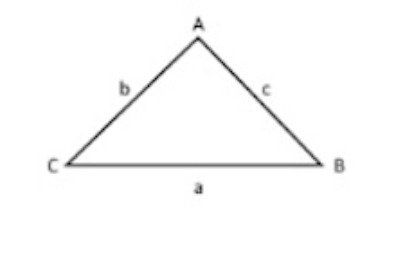# Formulas for Math on the ParaPro Test: Part 2—Geometry and Measurement

Geometry and measurement are areas of Math where students tend to struggle a lot. If you’re studying for the ParaPro Math Test, then you’re going to be asked about how to determine areas, perimeters, volumes… and how to teach them to your students, so you’ll need to dominate the formulas first.

That’s why we’ve prepared a formula chart with the geometry and measurement formulas you’ll need if you want to ace the ParaPro Test and teach the subject you love! You’ll also need these two formula charts for some questions:

Formulas for ParaPro Number Sense and Algebra Questions

Formulas for ParaPro Data Analysis Questions

Also, check out our mathematics study guide for more in-depth information about each formula. Ready to test your knowledge? Try our mathematics practice questions and flashcards.

## Geometry and Measurement Formulas for the ParaPro Test

Category Formula Symbols Comment
Geometry and
Measurement
$$Du = Su \cdot \dfrac{Du}{Su} = Su \cdot CF$$ $$Du =$$ Desired Unit
$$Su =$$ Starting Unit
$$CF =$$ Conversion Factor
Multiple Steps
may be needed
Geometry and
Measurement
$$P = 4 \cdot s$$ $$P =$$ Perimeter of a square
$$s=$$ side length

Geometry and
Measurement
$$P = 2l + 2w$$ $$P =$$ Perimeter of a rectangle
$$l =$$ length
$$w =$$ width

Geometry and
Measurement
$$P = s_1 + s_2 + s_3$$ $$P =$$ Perimeter of a triangle
$$s_n =$$ side length

Geometry and
Measurement
$$C = 2 \cdot \pi \cdot r = \pi \cdot d$$ $$C =$$ Perimeter of a circle
$$r =$$ radius
$$d =$$ diameter
$$\pi \approx 3.14$$

Geometry and
Measurement
$$s = r \cdot \theta$$ $$s =$$ arc length
$$r =$$ radius
$$\theta =$$ central angle (radians)

Geometry and
Measurement
$$A = s^2$$ $$A =$$ Area of a square
$$s =$$ side length

Geometry and
Measurement
$$A = l \cdot w$$ $$A =$$ Area of a rectangle
$$l =$$ length
$$w =$$ width

Geometry and
Measurement
$$A = \frac{1}{2}bh$$ $$A =$$ Area of a triangle
$$b =$$ base
$$h =$$ height

Geometry and
Measurement
$$A = \pi \cdot r^2$$ $$A =$$ Area of a circle
$$r =$$ radius

Geometry and
Measurement
$$A = h \cdot \dfrac{b_1 +b_2}{2}$$ $$A =$$ Area of a trapezoid
$$b_n =$$ base n
$$h =$$ height

Geometry and
Measurement
$$V = s^3$$ $$V =$$ Volume of a cube
$$s =$$ side length

Geometry and
Measurement
$$V = l\cdot w \cdot h$$ $$V =$$ Volume of a rectangular prism
$$l =$$ length
$$w =$$ width
$$h =$$ height

Geometry and
Measurement
$$V= \frac{4}{3} \cdot \pi \cdot r^3$$ $$V =$$ Volume of a sphere
$$r =$$ radius

Geometry and
Measurement
$$V = \pi \cdot r^2 \cdot h$$ $$V =$$ Volume of a cylinder
$$r =$$ radius
$$h =$$ height

Geometry and
Measurement
$$V = \frac{1}{3} \cdot \pi \cdot r^2 \cdot h$$ $$V =$$ Volume of a cone
$$r =$$ radius
$$h =$$ height

Geometry and
Measurement
$$V = \frac{1}{3} lwh$$ $$V =$$ Volume of a pyramid
$$l =$$ length
$$w =$$ width
$$h =$$ height

Geometry and
Measurement
$$SA = \Sigma A_{fi}$$ $$SA =$$ Surface Area of a Prism
$$A_{fi} =$$ Area of face i

Geometry and
Measurement
$$SA = \Sigma A_{fi}$$ $$SA =$$ Surface Area of a Pyramid
$$A_{fi} =$$ Area of face i

Geometry and
Measurement
$$SA = 2B + C\cdot h$$ $$SA =$$ Surface Area of a Cylinder
$$B =$$ Area of the Base
$$C =$$ Circumference of the Base
$$h =$$ height

Geometry and
Measurement
$$SA = B + \frac{1}{2} \cdot C \cdot l$$ $$SA =$$ Surface Area of a Cone
$$B =$$ Area of the Base
$$C =$$ Circumference of the Base
$$l =$$ slant height

Geometry and
Measurement
$$SA = 4 \pi r^2$$ $$SA =$$ Surface Area of a Sphere
$$r =$$ radius of the sphere

Geometry and
Measurement
$$d = \sqrt{(y_2 - y_1)^2 + (x_2 - x_1)^2}$$ $$d =$$ distance between two points
$$y_n =$$ y score at point n
$$x_n =$$ x score at point n

Geometry and
Measurement
$$a^2 + b^2 = c^2$$ $$a,b =$$ legs of a right triangle
$$c =$$ hypotenuse of a right triangle

Geometry and
Measurement
$$(x-h)^2 + (y - k)^2 = r^2$$ $$(h,k) =$$ center of a circle
$$r =$$ radius
Standard form
of a circle
Geometry and
Measurement
$$x^2 + y^2 + Ax + By + C = 0$$ $$x,y =$$ variables
$$A, B, C =$$ constants
General form
of a circle
Geometry and
Measurement
$$\cos ^2 \theta + \sin ^2 \theta = 1$$
Geometry and
Measurement
$$\sin 2\theta = 2 \cdot \sin \theta \cdot \cos \theta$$
Geometry and
Measurement
$$\cos 2 \theta = \cos ^2 \theta - \sin ^2 \theta = 2 \cos ^2 \theta - 1$$
Geometry and
Measurement
$$\tan (2\theta) = \dfrac{ 2 \tan \theta}{1-\tan ^2 \theta}$$

Formulas Requiring Graphics$\dfrac{a}{\sin A} = \dfrac{b}{\sin B} = \dfrac{c}{\sin C}$ $a^2= b^2 +c^2 - 2bc \cos A$ $b^2 = a^2 + c^2 - 2ac \cos B$ $c^2 = a^2 + b^2 - 2ab \cos C$